# 切片的内部实现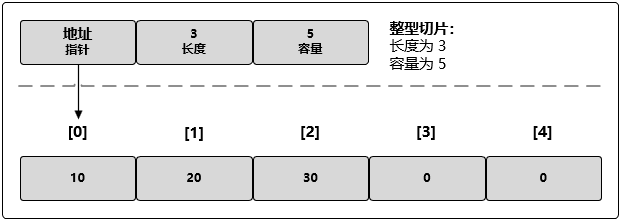# 切片的创建和初始化

// 创建一个整型切片
// 其长度和容量都是 5 个元素
slice := make([]int, 5)

// 创建一个整型切片
// 其长度为 3 个元素，容量为 5 个元素
slice := make([]int, 3, 5)

// 创建一个整型切片
// 使其长度大于容量
myNum := make([]int, 5, 3)

len larger than cap in make([]int)

// 创建字符串切片
// 其长度和容量都是 3 个元素
myStr := []string{"Jack", "Mark", "Nick"}
// 创建一个整型切片
// 其长度和容量都是 4 个元素
myNum := []int{10, 20, 30, 40}

// 创建字符串切片
// 使用空字符串初始化第 100 个元素
myStr := []string{99: ""}

// 创建有 3 个元素的整型数组
myArray := int{10, 20, 30}
// 创建长度和容量都是 3 的整型切片
mySlice := []int{10, 20, 30}

# nil 和空切片

// 创建 nil 整型切片
var myNum []int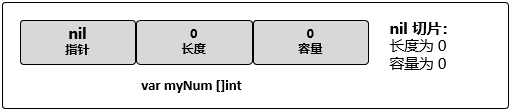// 使用 make 创建空的整型切片
myNum := make([]int, 0)
// 使用切片字面量创建空的整型切片
myNum := []int{}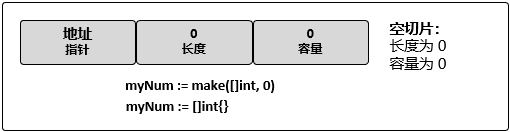# 为切片中的元素赋值

// 创建一个整型切片
// 其容量和长度都是 5 个元素
myNum := []int{10, 20, 30, 40, 50}
// 改变索引为 1 的元素的值
myNum  = 25

# 通过切片创建新的切片

slice[i:j]
slice[i:j:k]

slice[i:]  // 从 i 切到最尾部
slice[:j]  // 从最开头切到 j(不包含 j)
slice[:]   // 从头切到尾，等价于复制整个 slice

// 创建一个整型切片
// 其长度和容量都是 5 个元素
myNum := []int{10, 20, 30, 40, 50}
// 创建一个新切片
// 其长度为 2 个元素，容量为 4 个元素
newNum := slice[1:3]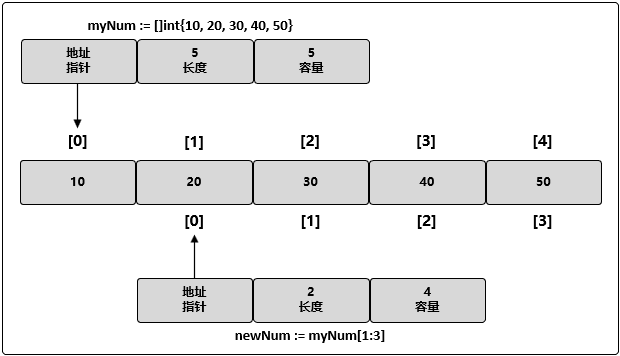// 修改 newNum 索引为 1 的元素
// 同时也修改了原切片 myNum 的索引为 2 的元素
newNum = 35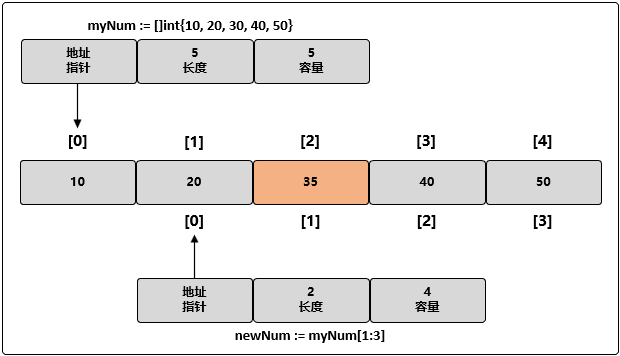// 修改 newNum 索引为 3 的元素
// 这个元素对于 newNum 来说并不存在
newNum = 45

panic: runtime error: index out of range

# 切片扩容

myNum := []int{10, 20, 30, 40, 50}
// 创建新的切片，其长度为 2 个元素，容量为 4 个元素
newNum := myNum[1:3]
// 使用原有的容量来分配一个新元素
// 将新元素赋值为 60
newNum = append(newNum, 60)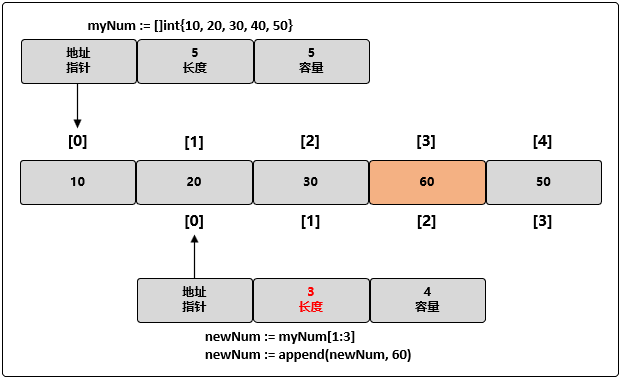// 创建一个长度和容量都是 4 的整型切片
myNum := []int{10, 20, 30, 40}
// 向切片追加一个新元素
// 将新元素赋值为 50
newNum := append(myNum, 50)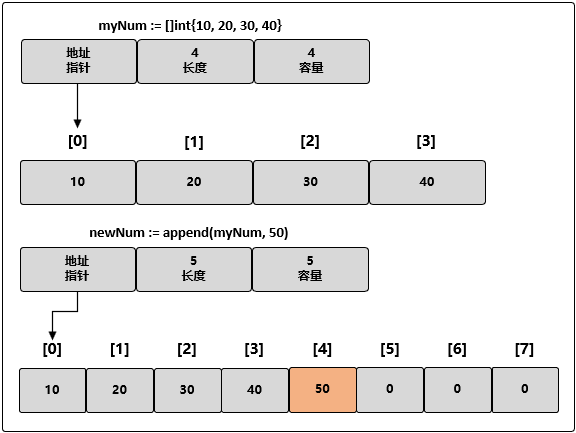# 限制切片的容量

// 创建长度和容量都是 5 的字符串切片
fruit := []string{"Apple", "Orange", "Plum", "Banana", "Grape"}

// 将第三个元素切片，并限制容量
// 其长度为 1 个元素，容量为 2 个元素
myFruit := fruit[2:3:4]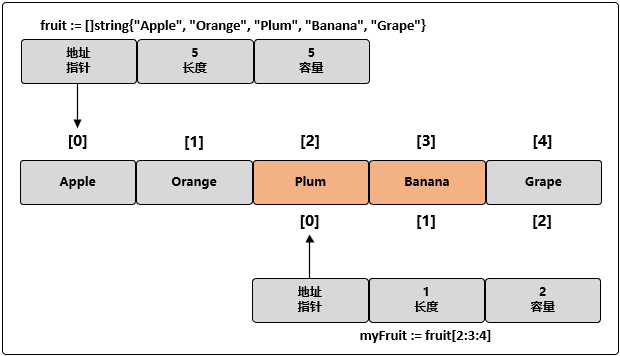myFruit := fruit[2:3:6]
panic: runtime error: slice bounds out of range

myFruit := fruit[2:3:3]
// 向 myFruit 追加新字符串
myFruit = append(myFruit, "Kiwi")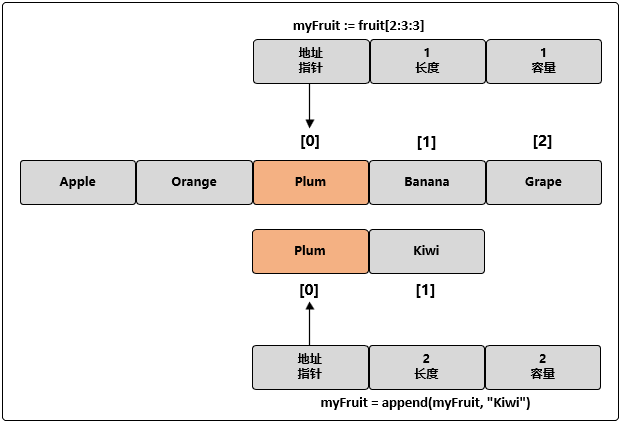// 创建两个切片，并分别用两个整数进行初始化
num1 := []int{1, 2}
num2 := []int{3, 4}
// 将两个切片追加在一起，并显示结果
fmt.Printf("%v\n", append(num1, num2...))

[1 2 3 4]

# 遍历切片

myNum := []int{10, 20, 30, 40, 50}
// 迭代每一个元素，并显示其值
for index, value := range myNum {
fmt.Printf("index: %d value: %d\n", index, value)
}

index: 0 value: 10
index: 1 value: 20
index: 2 value: 30
index: 3 value: 40
index: 4 value: 50

myNum := []int{10, 20, 30, 40, 50}
// 修改切片元素的值
// 使用空白标识符(下划线)来忽略原始值
for index, _ := range myNum {
myNum[index] += 1
}
for index, value := range myNum {
fmt.Printf("index: %d value: %d\n", index, value)
}

index: 0 value: 11
index: 1 value: 21
index: 2 value: 31
index: 3 value: 41
index: 4 value: 51

myNum := []int{10, 20, 30, 40, 50}
// 从第三个元素开始迭代每个元素
for index := 2; index < len(myNum); index++ {
...
}

# 切片间的拷贝操作

Golang 内置的 copy() 函数可以将一个切片中的元素拷贝到另一个切片中，其函数声明为：

func copy(dst, src []Type) int

num1 := []int{10, 20, 30}
num2 := make([]int, 5)
count := copy(num2, num1)
fmt.Println(count)
fmt.Println(num2)

3
[10 20 30 0 0]

3 表示拷贝成功的元素个数。

# 把切片传递给函数

myNum := make([]int, 1e6)
// 将 myNum 传递到函数 foo()
slice = foo(myNum)
// 函数 foo() 接收一个整型切片，并返回这个切片
func foo(slice []int) []int {
...
return slice
}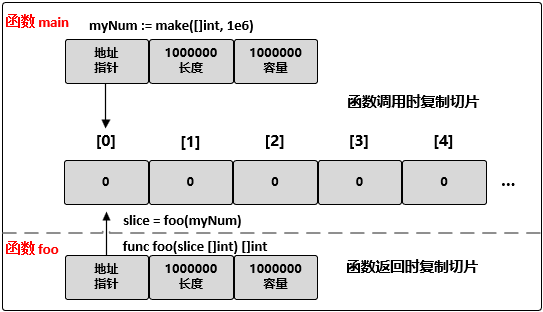# 总结

The Go Programming Language Specification
《Go 语言实战》
《Go语言编程入门与实战技巧》
Go基础系列：Go slice详解

posted @ 2019-04-17 07:52 sparkdev 阅读(...) 评论(...) 编辑 收藏